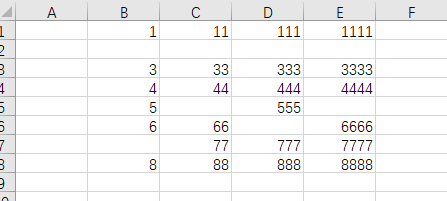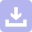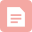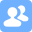# 1 要解决的问题：删除某列中的空单元格/空行

## 1.1 需求分析

1. 删除1列的空行（本文要做的）
2. 删除整个区域内的空行
3. 删除整个区域内的空格（这个一般很少有这种需求，用处不大----可用currentregion .specialcells()解决
4. 下面是原始数据，下面看看如何处理# 2 如果是删除全表/某区域的空单元格, 直接在当前列删除

### 简洁代码：删除区域内所有空单元格 cells ---实际需要少，so用处不大

• 删除，表格页面内使用区域的空格所在的行
• 局限性就是表格的上方，左边还会存在一些空行空列，不过这个手动删下就可以了
• 这个只是删除了空的cells 并不是删除了空行
Sub 删空单元格()

ActiveSheet.UsedRange.SpecialCells(xlCellTypeBlanks).Delete

End Sub

# 3 如果是删一列的空行

## 3.1 代码1：基础代码，假设确认知道这列的长度，关键是倒着删

• 熟悉 isempty() 或者用 if   xxxx=""
• 删除单元格后，要设置属性 shift:=xlup等
• 需要倒着删除，否则会因为一边删除行，一遍重新排列有问题
Sub 删一列的空行()

For i = 20 To 1 Step -1    '需要倒着删除
If IsEmpty(Cells(i, 1)) Then
Cells(i, 1).Delete shift:=xlUp
End If
Next i

End Sub

### 3.2 改进版: 先查这一列的非空最大行数

Sub jackma_delete_row()

For i = Range("c65536").End(xlUp).Rows To 1 Step -1

If IsEmpty(Cells(i, 3)) Then
rows(i).Delete shift:=xlUp
End If

Next i

End Sub

### 3.3 也可以不用 isempty()  函数，直接判断  if cells()=""

• 直接判断 if cells(i,j) =""
• 这种删除整行不合适  rows(i) .delete，会影响其他列的元素
Sub ponyma_del_row1()

For i = Range("c65536").End(xlUp).Row To 1 Step -1
If Cells(i, 3) = "" Then
Cells(i, 3).Delete
'      Rows(i).Delete
End If

Next i

End Sub


3.3 如果是要先删除前面的 重复行，可以正着查询。


'这次收到的需求是，要删掉相同的行里，前面的行
Sub del_test2()

Dim ws1 As Object
Dim ws2 As Object
Dim ws3 As Object

Set ws1 = Worksheets("sheet1")
Set ws2 = Worksheets("sheet2")
Set ws3 = Worksheets("sheet3")

'maxr1 = ws1.Range("a1").End(xlDown).Row

'删行后会导致行数变少
'End(xlDown) 最大的问题还是可能一列中间有空的，断了，查找不准
'反过来查比较准, range("a9999").end(xlup).row

maxr1 = 999       '其实经验发现，把这些配置值放表里出错可能性大，要么弄个控件，要么干脆放代码里

For i = 1 To maxr1

If WorksheetFunction.CountIf(ws1.Columns(1), ws1.Cells(i, 1)) > 1 Then
ws1.Rows(i).Delete
End If

Next

Debug.Print "done"

End Sub

## 4 不动原数据，将非空数据放到另外一列

### 4.1 错误版：

• 要明白“写入列”的循环数，明显和“输出列”的循环数 应该不同！
• 这么写相当于 输出列 那行为空的时候，只是不往 写入列写，所以没覆盖，也是空的
Sub jackma_delete_row2()

For i = 1 To Range("c65536").End(xlUp).Rows

If Not IsEmpty(Cells(i, 3)) Then
Cells(i, 9) = Cells(i, 3)
End If

Next i

End Sub


### 4.2 正确版： 写入列/输出列，应该是单独的循环变量！

Sub jackma_delete_row2()
k = 1

For i = 1 To Range("c65536").End(xlUp).Rows

If Not IsEmpty(Cells(i, 3)) Then
Cells(k, 9) = Cells(i, 3)
k = k + 1
End If

Next i

End Sub


# 5 先写入数组array中，再写到其他地方，据说这样能大幅提高速度！

### 第1版：局限性很大，没有自动查这列的 元素个数，以及 最大非空行是多少

Sub 删除空格4()
Dim arr1()                          '定义了一个数组，并且是动态数组，因为没指定大小

ReDim arr1(11)                      '动态数组，使用前必须重新redim，数组大小
j = 0                              'j=1开始，不会越界，但arr1(0)为空，因为赋值跳过了它
For i = 1 To 11 Step 1
If Not IsEmpty(Cells(i, 1)) Then
arr1(j) = Cells(i, 1)
j = j + 1
End If
Next i

For j = 0 To UBound(arr1())
Cells(j + 1, 9) = arr1(j)      '单元格得从1开始，arr()，默认得从0开始，但可以改
Next j

End Sub

### 第2版重写

• 注意debug.print用来监测的时候，需要注意，放在循环的位置，尤其是在k=K+1这种变化时，和放在哪个for循环之内外！

Sub ponyma_array22()
Dim arr1()  '当数组定义，且默认开始的index为0！ preserve时需要有0的index
'dim arr1 当变量定义

k = 1
m = 1

ReDim arr1(0 To Application.WorksheetFunction.CountA(Range("c:c")))

For i = 1 To Range("c65536").End(xlUp).Row Step 1
If Cells(i, 3) <> "" Then

Debug.Print Cells(i, 3)
arr1(k) = Cells(i, 3)
Debug.Print arr1(k)
k = k + 1
'          Debug.Print arr1(k),写在这里问题1：k已经变了，下一个k还没赋值为空，2最后的k越界
'          循环是很精巧的，放的地方很讲究，放得不对，就错误百出
'           Debug.Print arr1(k) 如果放在k=K+1 后，就看起来没打印出东西，因为都打印的arr1的空元素

End If
Next i

For j = 1 To UBound(arr1(), 1)
Cells(m, 10) = arr1(j)
m = m + 1
Next j

End Sub

### 第3版

Option Explicit

Sub ponyma1()

Dim arr1()
Dim k1, k2, k
Dim i, j
k1 = WorksheetFunction.CountA(Range("a:a"))
k2 = Range("a65536").End(xlUp).Row
Debug.Print "这列非空数据个数k1=" & k1
Debug.Print "这列最后1个有数据的行数k2=" & k2

'arr1(0) = 1
'ReDim Preserve arr1(1, k)
'这样会越界，因为你需要preserve数据。但是index系不符合
'但是，如果不preserve 就无所谓
'或者虽然 dim arr1() 是动态数据从index0开始，但是arr1()一直为空,preserve也不会出现index越界问题

ReDim Preserve arr1(1 To k1)
'ReDim Preserve arr1(1, k1) 这样就会越界。。。因为语法是2维数组了！
'ReDim Preserve arr1(1 to k1) 这样就对的

k = 1
For i = 1 To k2
If Cells(i, 1) <> "" Then
arr1(k) = Cells(i, 1)
Debug.Print arr1(k)
k = k + 1
End If
Next i下载
VBA_EXCEL删除方法
04-18下载
VBA示例之 删除表格中使范围内所有空白单元格
10-26博客
VBA删掉某中含有空值
06-091413博客
Excel VBA删除行、单元格
07-213418博客
VBA-常函数集锦-13-Excel多行或多删除

08-132万+博客
Excel·VBA指定条件删除整行整

03-153201博客
c判断数组是否为空_VBA实验1VBA 删除某列空单元格3方法删除转移其他方法数组方法...
11-28205博客

01-12499博客
VBA EXCEL删除方法
09-081717博客
word、wps中使vba删除所有表格指定
10-22829帖子
excel vba如何删除选定区域内不等于指定内容单元格所在
07-15博客

01-141690博客
cricheditview实现语高亮和行号_VBA实践(7)---分区域删除单元格实现内容连续...
11-2550博客

06-112232博客

11-101024博客
VBA代码删除字符串里所有空格邮件地址内空格专杀！
03-253059博客
VBA统一数字单位
01-28273博客
split多个空格视为一个_文本间有空格！手动删？太慢！VBA帮你删除前、后、中间所有空格...
12-03232博客

01-15732

### “相关推荐”对你有帮助么？

•非常没帮助
•没帮助
•一般
•有帮助
•非常有帮助¥2 ¥4 ¥6 ¥10 ¥20余额支付 (余额：-- )扫码支付获取中扫码支付点击重新获取扫码支付1.余额是钱包充值的虚拟货币，按照1:1的比例进行支付金额的抵扣。
2.余额无法直接购买下载，可以购买VIP、C币套餐、付费专栏及课程。余额充值